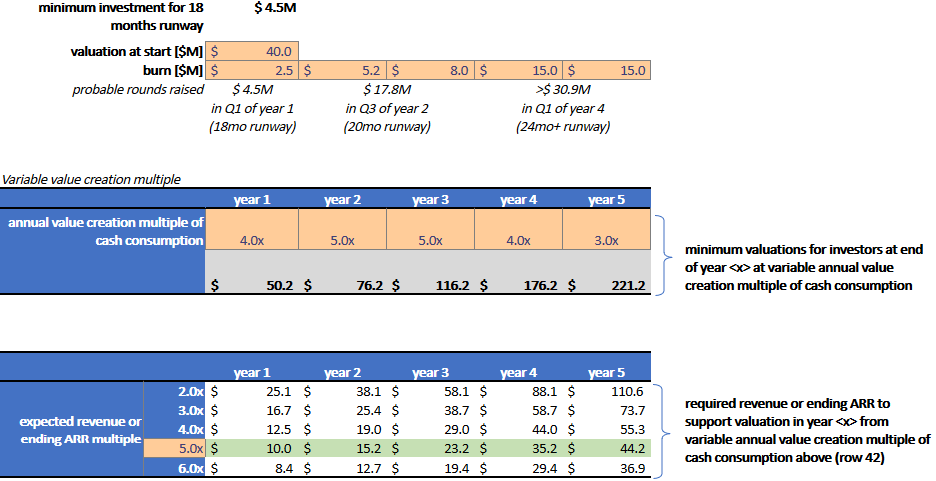# Fred Wilson’s “Return on Burn” (Excel Worksheet Attached)

Minimum valuations and their required revenue at fixed value-creation-multiple of cash consumption. In the last blog post I wrote about Fred Wilson’s excellent insight into value creation relative to cash burn. I got a lot of questions about it so here is an Excel spreadsheet for you to download and play with — let me know if you find an error, I might have gotten it wrong. Also: this is a rule-of-thumb approach about expectations and trajectories. Do not take this literally. Another way to look at this Excel worksheet is for experimentation: What happens if your cash burn suddenly doubles?

## Section 1: Calculate your annual value creation as a multiple of cash burn

You enter revenue and revenue multiples and get your value creation. You enter your anticipated burn rate and get the resulting value-creation-to-burn ratio. Below is a table with fixed value-creation-to-cash-burn ratios and the maximum cash you could burn to achieve these multiples.

## Section 2: Calculate minimum valuations and their required revenue at a fixed value-creation-multiple of your cash burnExcel Worksheet: Approach 2 — Minimum valuations and their required revenue at fixed value-creation-multiple of cash consumption

You enter your current valuation and expected cash burn over the next couple of years. Below is a very rough estimate of the rounds you’re going to raise (edit these if you want).

The first table assumes a fixed value-creation-to-burn rate ratio over the next couple of years (here 2.0x to 6.0x). given your estimated cash burn, an investor might want to see these minimum valuations in each of the subsequent years.

The second table lets your enter some revenue multiples on the top for each of the years (here assuming 5.2x flat) and see what the required ending revenue or ARR would be. For example, assuming a 5.0x value-creation-to-cash-burn ratio, and a 5.2x LTM revenue multiple at the end of year 3, you would need to create \$22.8 million in revenue to support a \$118.7 million valuation, based on the assumption that you will have a cash burn of \$2.5, \$5.2, and \$8.0 million in years 1,2,3.

Again: if you are using NTM multiples, then calculate your LTM multiples yourself. I think you’ll find the mechanics and insights interesting once you start playing around with this. This table does not mean that you’re actually going to find a buyer. Nor does it guarantee that you’ll find a follow-on investor at that valuation and revenue — that will depend on many other factors.

## Section 3: Calculate minimum valuations and their required revenue at variable value-creation-multiples of cash burnExcel Worksheet: Approach 3 — Minimum valuations and their required revenue at variable value-creation-multiples of cash consumption

You enter your valuation at start and your estimated cash burn  over the next couple of years. Below is a very rough estimate of the rounds you’re going to raise (edit these if you want).

The first table lets you assume different value-creation-to-cash-burn ratio over the years (here starting with 4.0x and ending with 3.0x) . The lower bottom line of that table shows the mininum valuation for investors, based on your cash burn and ratio assumptions.

The second table lets you enter an expected LTM revenue or ARR multiple (here from 2.0x to 6.0x). You can calculate your own NTM multiples if you want instead. each column shows the required revenue or ending ARR to support the valuation from the first table. For example: At a 5.0x LTM revenue multiple, you would need to show \$23.2 million in revenue at the end of year 3 to support a valuation of \$116.2 million, based on the progression of value-creation-to-cash-burn ratios of 4.0x/5.0x/5.0x in year 1/2/3, and your projected cash burn in these years.